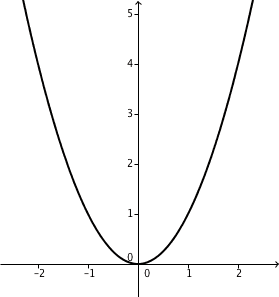# Track & TraceThe ends of a stiff bar $\overline{AB}$ of length 4 slide freely inside a parabolic track (specifically, the parabola $y=x^2$). As they do, the midpoint $M$ of that bar traces a curve. Find the area of the region between the parabola and the curve traced by $M$.

Assume that the bar slides infinitely in both directions.

×